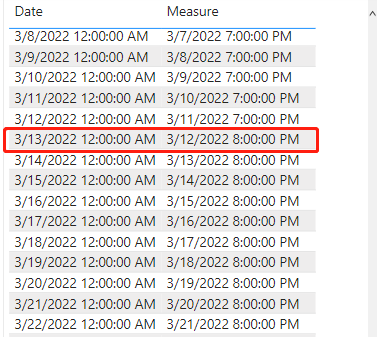cancel
Showing results for
Did you mean:Frequent Visitor

## Measure to Convert UTC to local time, accounting for daylight savings

Hello. I am creating a dashboard using a direct query to Dataverse, with automatic page refresh when a change is detected. Because I am using direct query, I can do very little transformation in Power Query. And because I am using automatic page refreshes, I cannot use calculated tables or columns because then the visuals do not refresh when a change is detected.

As a result, most of my table columns in the table visuals are coming from measures. I have one measure to convert the time from UTC to local (local date = Selectedvalue(table[date/time]) - TIME(5,0,0).

However, I need a way to condition this statement so if the date falls within daylight savings time, the measure will only subtract 4 hours. Is this possible? I found several articles on how to do this in power query, but I cannot use power query for this.

1 ACCEPTED SOLUTIONCommunity Champion

hi @mll92

tried to write a measure like this:

``````Measure =
VAR _table =
ALL(DateTable[Date]),
"Day",
WEEKDAY(DateTable[Date], 2),
"Month",
MONTH(DateTable[Date])
)
VAR _table1 =
FILTER(
_table,
[Month]=3&&[Day] = 7
)
VAR _shiftdate =
EXCEPT(
TOPN(
2,
_table1,
DateTable[Date],
ASC
),
TOPN(
1,
_table1,
DateTable[Date],
ASC
)
)
VAR _shiftdate2 = MINX(_shiftdate, [Date])
RETURN
IF(
MAX([Date])<_shiftdate2,
MAX([Date]) - TIME(5,0,0),
MAX([Date]) - TIME(4,0,0)
)``````

When plot a table visual with the date column, it worked like this:4 REPLIES 4Frequent Visitor

On the second Sunday in March, the difference between UCT and local will change from -5 hours to -4 hours. Then return to -5 on the first Sunday in NovemberCommunity Champion

hi @mll92

tried to write a measure like this:

``````Measure =
VAR _table =
ALL(DateTable[Date]),
"Day",
WEEKDAY(DateTable[Date], 2),
"Month",
MONTH(DateTable[Date])
)
VAR _table1 =
FILTER(
_table,
[Month]=3&&[Day] = 7
)
VAR _shiftdate =
EXCEPT(
TOPN(
2,
_table1,
DateTable[Date],
ASC
),
TOPN(
1,
_table1,
DateTable[Date],
ASC
)
)
VAR _shiftdate2 = MINX(_shiftdate, [Date])
RETURN
IF(
MAX([Date])<_shiftdate2,
MAX([Date]) - TIME(5,0,0),
MAX([Date]) - TIME(4,0,0)
)``````

When plot a table visual with the date column, it worked like this:Frequent Visitor

Thank you!Community Champion

hi @mll92

how is daylight savings time is defined in your region?